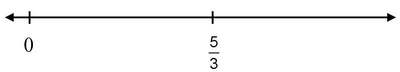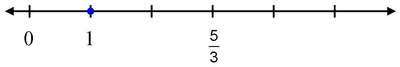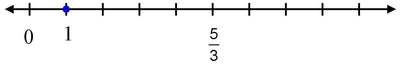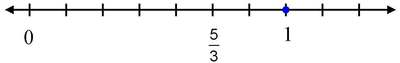# Find 1 Starting from 5/3, Assessment Variation

Alignments to Content Standards: 3.NF.A.2

The number line below shows two numbers, 0 and $\frac53$.Where is 1 on this number line?

## IM Commentary

This task is part of a joint project between Student Achievement Partners and Illustrative Mathematics to develop prototype machine-scorable assessment items that test a range of mathematical knowledge and skills described in the CCSSM and begin to signal the focus and coherence of the standards.

This is the third of three summative assessment tasks for 3.NF.2 that progress in difficulty. Each requires that students "understand a fraction as a number on the number line" and "represent fractions on a number line diagram." Part (a) of the standard is about representing unit fractions and part (b) is about representing fractions in terms of unit fractions. The first task involves (a); the second two involve both (a) and (b) at different levels of complexity. The tasks require attention to the whole when thinking about fractions; on a number line, the whole is the interval from 0 to 1.

### Cognitive Complexity

##### Mathematical Content

The three tasks increase in complexity:

##### Mathematical Practices

Proficiency with the number line is an important component of students’ ability to reason abstractly and quantitatively (MP 2).

While the number line is an important tool, these tasks do not ask for strategic use of tools (MP 5) because the number line is provided. An item calling for MP 5 might be one that is easy if the student uses a number line, but difficult if not.

These tasks give students an opportunity to look for and make use of structure (MP 7), namely, the partitioning of the unit interval.

##### Linguistic Demand

The linguistic demand is low.

##### Stimulus Material

The stimulus material is not complex, consisting of only a short written prompt and a number line.

##### Response Mode

The response mode might be unfamiliar to students. However, they can mess around without penalty and there is a reset option. The spacing on the selector for the tick marks should be wide enough so that students can easily pick the refinement they want. The point has a fairly large radius relative to the scale of the number line.

• All of these can be turned into multiple choice tasks, as in, for example, 3.NF Find 1 starting from 5/3. However, the multiple choice variation is less complex because the technology enhanced version asks students to construct a number line rather than select one.
• An instructional extension would be a task asking students to "Find 2/3 starting from 1/4." This would be more complex because it requires juggling two partitions of the unit interval, into fourths and then thirds.
• Here is a multiple choice version of this task:

The number line below shows two numbers, 0 and $\frac53$.Where is 1 on this number line?## Attached Resources

• Geogebra sketch for find 1 from 5/3
• ## Solution

This is a one point task; the correct answer is shown in the video. Students will get full credit as long as the point touches the correct tick mark at all.